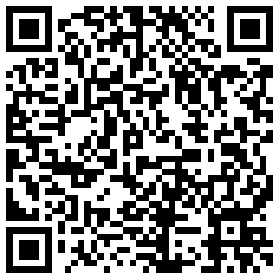# 惠普宣布全球召回7万块笔记本锂离子电池

62940^^AXV^^^^ 65033^^B7U^^^^

65033^^B7V^^^^

65033^^BGU^^^^ 65035^^B7U^^^^

65035^^B7V^^^^

65035^^BGU^^^^

65035^^BGV^^^^ 67059^^V8U^^^^

67059^^V8V^^^^